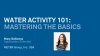Let’s consider the reduction in vapor pressure. We can calculate the change in energy that accompanies a change in pressure using the first law of thermodynamics. If we let the symbol U represent the energy in a system and calculate the change in U that occurs when we change the volume, at constant pressure (we assume no heat is added or removed) we can writeEquation 1

dU represents a small change in energy, and dV represents a small change in volume. The relationship between pressure and volume, called the ideal gas law, isEquation 2

where n is the number of moles of gas, R is a constant, known as the gas constant (8.31 J/mol K) and T is the temperature of the gas in kelvins. We can differentiate the ideal gas law to get dVEquation 3

Combining this with the first law we getEquation 4

Now, the energy required to go from the vapor pressure of the pure water in the glass, which we call the saturation vapor pressure or p0, to the vapor pressure of the water in the sponge isEquation 5

The ratio p /p0 is called the water activity (aw) when we are talking about the water in the sponge or water in foods or other solids or liquids. We call it the relative humidity when we apply it to water in the air and sometimes multiply it by 100 to express it as a percent. The ratio U/n is the energy per mole of water and is called the water potential, with the symbol Ψ. Water potential has units of Joules/mole. With this substitution, we finally arrive at the equation relating the energy of the water in the sponge and its water activityEquation 6

The equation means that we can express the energy state of the water in a product either as a water potential or as a water activity. Some fields of science use water potential and others use water activity. Some also use freezing point depression or osmolality, but these are all equivalent concepts. There are advantages and disadvantages to each, but the important thing to understand is that all are measures of the energy state of the water and have a strong theoretical basis. Water activity is the measure most widely used in food science and engineering.

## Master the basics

Get the essentials of water activity condensed in this 20 minute webinar. You’ll learn:

-What water activity is
-How it’s different from moisture content
-Why it controls microbial growth
-How understanding water activity can help you control moisture in your product.## Get the complete picture

Learn everything about how water activity can increase your profit and improve product quality and safety—all in one place.

Download The Food Manufacturer’s Complete Guide to Water Activity—>

Learn how to predict shelf-life using water activity.

Download The Food Manufacturer’s Complete Guide to Shelf Life—>

## What is water activity?

Water activity is directly related to the microbial susceptibility of food products and is correlated with many of the reactions that end shelf life in foods. Learn more about the science that makes water …